Related Articles

# Exploratory Data Analysis in Julia

• Difficulty Level : Basic
• Last Updated : 12 Oct, 2020

Exploratory Data Analysis (EDA) is used for achieving a better understanding of data in terms of its main features, the significance of various variables, and the inter-variable relationships. The First step is to explore the dataset.

Methods to explore the given dataset in Julia:

1. Using data tables and applying statistics
2. Using Visual Plotting techniques on the data

#### Using data tables and applying statistics

Step 1: Install DataFrames Package

For using data tables in Julia, a data structure called Dataframe is used. DataFrame can handle multiple operations without compromising on Speed and Scalability.

Dataframes package can be installed using the following command:

```using Pkg
```

For data analysis, we have used the Iris dataset. It is easily available online.

Step 3: Import Necessary Packages and the Dataset

Let’s first import our DataFrames Package, CSV Package, and load the Iris.csv file of the data set:

## Julia

 `# Using the DataFrames Package``using DataFrames`` ` `# Using the CSV package``using CSV`` ` `# Reading the CSV file``Iris ``=` `CSV.read(“Iris.csv”)`

Output: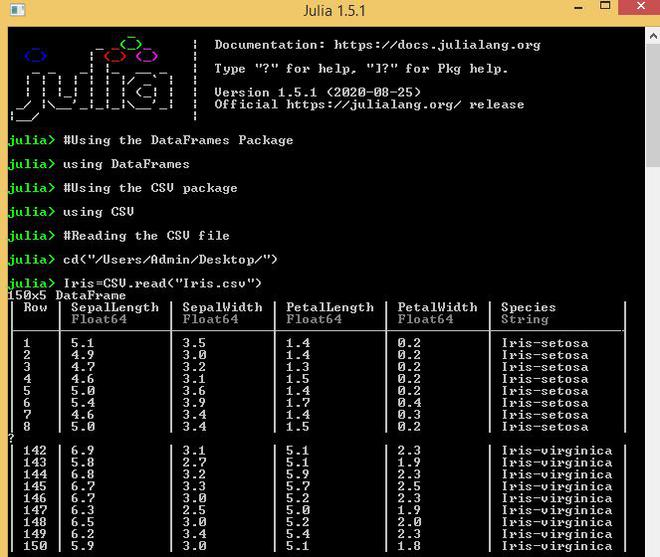Step 4: Quick Data Exploration

Preliminary exploration can be performed on the dataset such as identifying the shape of the dataset using the size function, list of columns using the names function, first n rows using the head function.

Example:

## Julia

 `# Using the DataFrames Package``using DataFrames`` ` `# Using the CSV package``using CSV`` ` `# Reading the CSV file``Iris ``=` `CSV.read(“Iris.csv”);`` ` `# Shape of Dataset``size(Iris)`` ` `# List of columns``names(Iris) `` ` `# First 10 rows``head(Iris, ``10``)`

Output: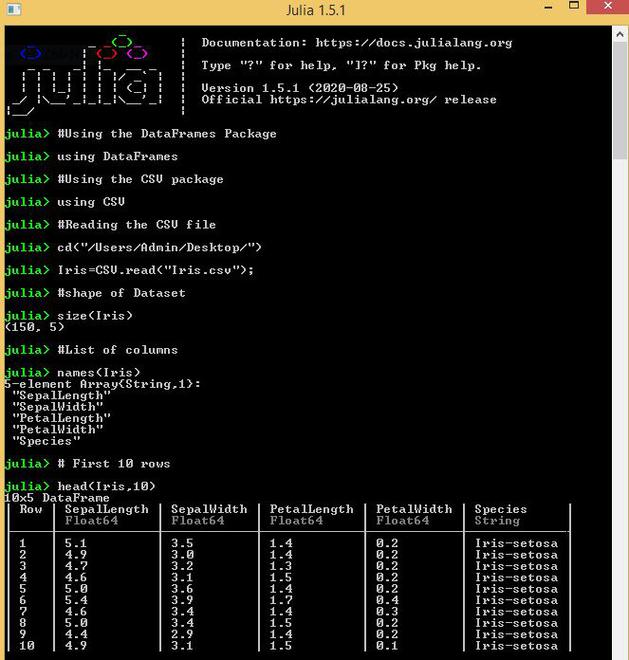#### Indexing in a dataframe

Dataframe_name[:column_name] is a basic indexing technique to access a particular column of the data frame.

Example:

## Julia

 `# Using the DataFrames Package``using DataFrames`` ` `# Using the CSV package``using CSV`` ` `# Reading the CSV file``Iris ``=` `CSV.read(“Iris.csv”);`` ` `# Access particular column of dataset``Iris[: SepalLength]`

Output: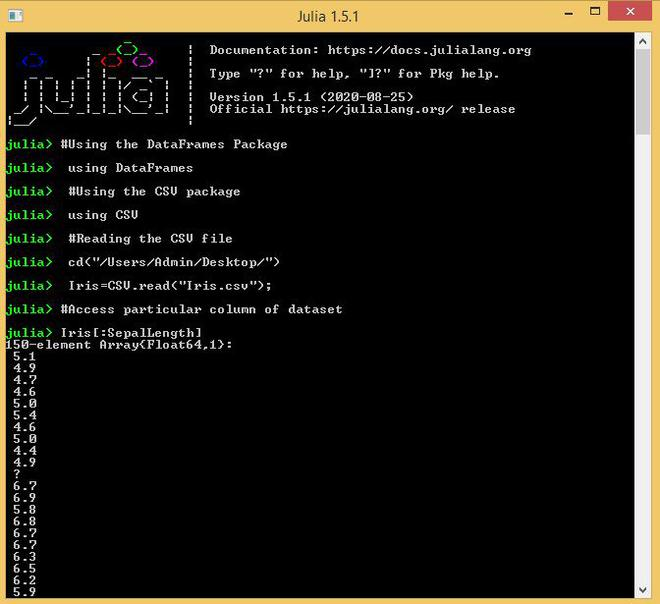#### Describe Function

Describe function is used to present the mean, median, minimum, maximum, quartiles, etc of the dataset.

Example:

## Julia

 `# Using the DataFrames Package``using DataFrames`` ` `# Using the CSV package``using CSV`` ` `# Reading the CSV file``Iris ``=` `CSV.read(“Iris.csv”);`` ` `# Using describe function ``# to get statistics of a dataset``describe(Iris)`` ` `# Using describe function to get``# statistics of a particular column in a dataset``describe(Iris, :``all``, cols``=``:SepalLength)`

Output: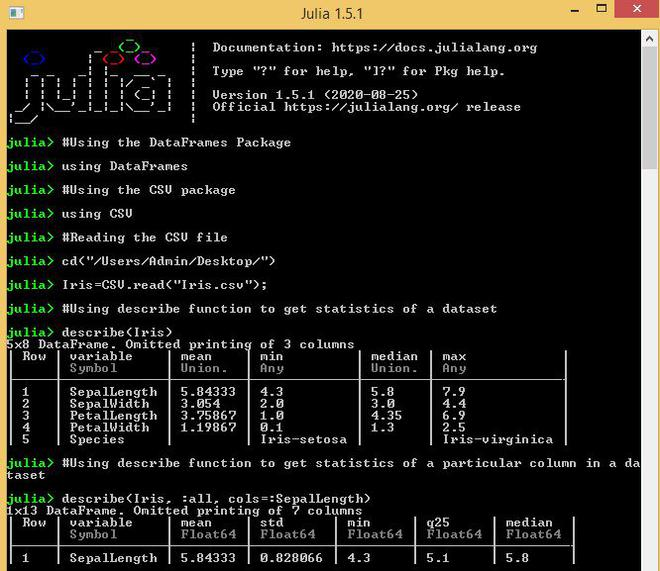#### Using Visual Plotting techniques on the data

Visual Exploration in Julia can be achieved with the help of various plotting libraries such as Plots, StatPlots, and Pyplot.

Plots: It is a high-level plotting package that interfaces other plotting packages called ‘back-ends‘. They behave like the graphics engines that generate the graphics. It has a simple and consistent interface.

StatPlots: It is a supporting package used with Plots package consisting of statistical recipes for various concepts and types.

PyPlot: It is used to work with mathplotlib library of Python in Julia.

The above-mentioned libraries can be installed using the following commands:

```Pkg.add(“Plots”)
```

#### Distribution analysis

The distribution of variables in Julia can be performed using various plots such as histogram, boxplot, scatterplot, etc.

Let’s start by plotting the histogram of SepalLength:

Example:

## Julia

 `# Using the DataFrames Package``using DataFrames``  ` `# Using the CSV package``using CSV``  ` `# Reading the CSV file``Iris ``=` `CSV.read(``"Iris.csv"``);`` ` `# Using Plots Package ``using Plots `` ` `# Plot Histogram``Plots.histogram(Iris[:SepalLength], ``                ``bins ``=` `50``, xlabel ``=` `"SepalLength"``, ``                            ``labels ``=` `"Length in cm"``)`

Output: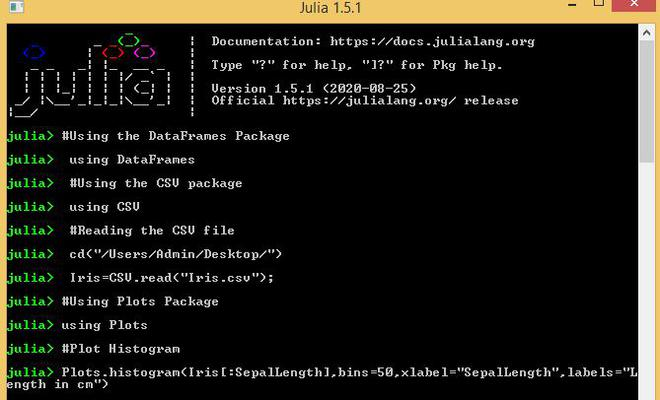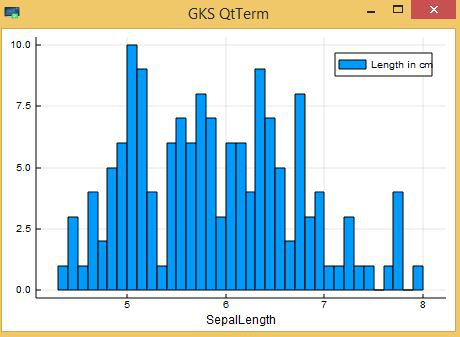Next, we look at box plots to understand the distributions. Box plot for SepalLength can be plotted by:

Example:

## Julia

 `# Using the DataFrames Package``using DataFrames``  ` `# Using the CSV package``using CSV``  ` `# Reading the CSV file``Iris ``=` `CSV.read(``"Iris.csv"``);`` ` `# Using Plots,StatPlots Package ``using Plots, StatPlots `` ` `# Plot Boxplot``Plots.boxplot(Iris[:SepalLength],``              ``xlabel ``=` `"SepalLength"``)`

Output: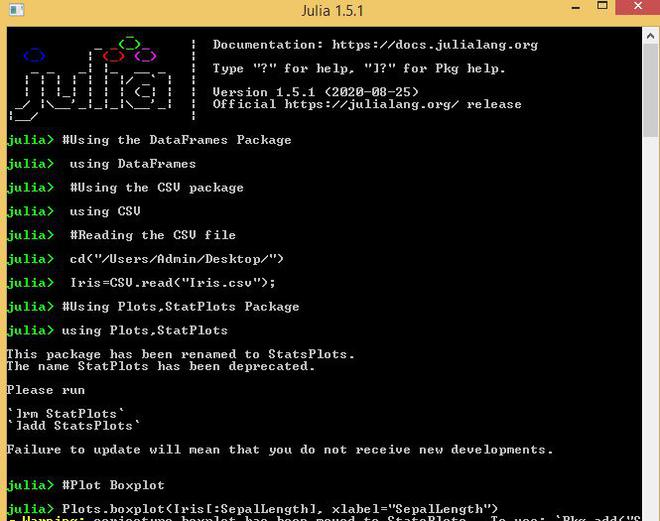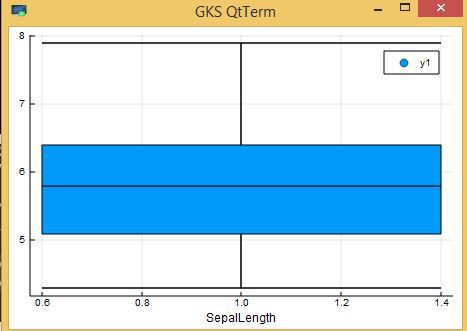Next, we look at Scatter plot to understand the distributions. Scatter plot for SepalLength can be plotted by:

Example:

## Julia

 `# Using the DataFrames Package``using DataFrames``  ` `# Using the CSV package``using CSV``  ` `# Reading the CSV file``Iris ``=` `CSV.read(``"Iris.csv"``);`` ` `# Using Plots package ``using Plots`` ` `# Plot Scatterplot``plot(Iris[:SepalLength], ``     ``seriestype ``=` `:scatter,``     ``title ``=` `"Sepal Length"``)`

Output: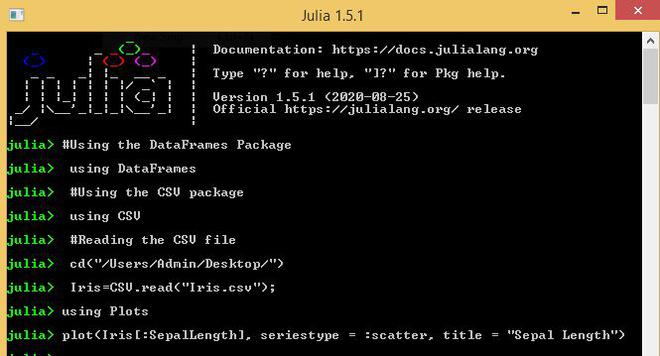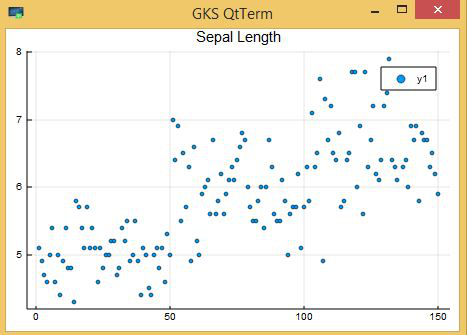My Personal Notes arrow_drop_up